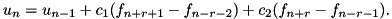A Survey of Gaussian Convolution Algorithms
Extended box filter Gaussian convolution

An improvement of box filtering with continuous selection of sigma. More...

## Detailed Description

An improvement of box filtering with continuous selection of sigma.

This code implements the extended box filter approximation of Gaussian convolution proposed by Gwosdek, Grewenig, Bruhn, and Weickert. The extended box filter is the recursive filterThe process to use these functions is the following:

1. ebox_precomp() to precompute coefficients for the convolution
2. ebox_gaussian_conv() or ebox_gaussian_conv_image() to perform the convolution itself (may be called multiple times if desired)
Example
buffer = (num *)malloc(sizeof(num) * N);
free(buffer);
Reference
• P. Gwosdek, S. Grewenig, A. Bruhn, J. Weickert, "Theoretical Foundations of Gaussian Convolution by Extended Box Filtering," International Conference on Scale Space and Variational Methods in Computer Vision, pp. 447-458, 2011. http://dx.doi.org/10.1007/978-3-642-24785-9_38

## Data Structures

struct  ebox_coeffs_
Coefficients for extended box filter Gaussian approximation. More...

## Typedefs

typedef struct ebox_coeffs_ ebox_coeffs
Coefficients for extended box filter Gaussian approximation.

## Functions

static void ebox_filter (num *dest, long dest_stride, const num *src, long src_stride, long N, long r, num c_1, num c_2)
Perform one pass of extended box filtering. More...

void ebox_precomp (ebox_coeffs *c, double sigma, int K)
Precompute coefficients for extended box filtering. More...

void ebox_gaussian_conv (ebox_coeffs c, num *dest, num *buffer, const num *src, long N, long stride)
Extended box filtering approximation of Gaussian convolution. More...

void ebox_gaussian_conv_image (ebox_coeffs c, num *dest, num *buffer, const num *src, int width, int height, int num_channels)
Extended box filtering approximation of 2D Gaussian convolution. More...

## Function Documentation

 static void ebox_filter ( num * dest, long dest_stride, const num * src, long src_stride, long N, long r, num c_1, num c_2 )
static

Perform one pass of extended box filtering.

Parameters
 dest destination array dest_stride stride between successive samples of dest src_stride src array (must be distinct from dest) src_stride stride between successive samples of src N number of samples r radius of the inner box c_1 weight of the outer box c_2 weight of the inner box

This routine performs one pass of extended box filtering with inner box radius `r`,Note
The computation is out-of-place, `src` and `dest` must be distinct.

Definition at line 69 of file gaussian_conv_ebox.c.

 void ebox_gaussian_conv ( ebox_coeffs c, num * dest_data, num * buffer_data, const num * src, long N, long stride )

Extended box filtering approximation of Gaussian convolution.

Parameters
 c ebox_coeffs created by ebox_precomp() dest_data destination array buffer_data array with space for at least N samples src input, overwritten if src = dest_data N number of samples stride stride between successive samples

This routine performs the extended box filtering approximation of Gaussian convolution by multiple passes of the extended box filter. The filtering itself is performed by ebox_filter().

Since ebox_filter() requires that the source and destination are distinct, the iteration alternates the roles of two arrays. See box_gaussian_conv() for more detailed discussion.

The convolution can be performed in-place by setting `src` = `dest_data` (the source array is overwritten with the result). However, `buffer_data` must be distinct from `dest_data`.

Definition at line 117 of file gaussian_conv_ebox.c.

 void ebox_gaussian_conv_image ( ebox_coeffs c, num * dest, num * buffer, const num * src, int width, int height, int num_channels )

Extended box filtering approximation of 2D Gaussian convolution.

Parameters
 c ebox_coeffs created by ebox_precomp() dest destination image buffer array with at least max(width,height) samples src input image, overwritten if src = dest width image width height image height num_channels number of image channels

Similar to ebox_gaussian_conv(), this routine approximates 2D Gaussian convolution with extended box filtering.

The convolution can be performed in-place by setting `src` = `dest` (the source array is overwritten with the result). However, `buffer` must be be distinct from `dest`.

Definition at line 185 of file gaussian_conv_ebox.c.

 void ebox_precomp ( ebox_coeffs * c, double sigma, int K )

Precompute coefficients for extended box filtering.

Parameters
 c ebox_coeffs pointer to hold precomputed coefficients sigma Gaussian standard deviation K number of filtering passes

This routine precomputes the coefficients for extended box filtering Gaussian convolution approximation.

Definition at line 35 of file gaussian_conv_ebox.c.